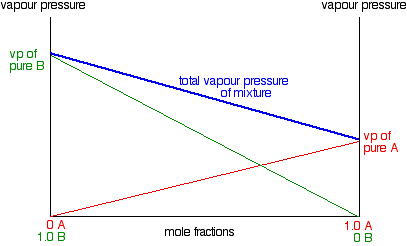# What type of phase diagram should I draw if more than one pure component present?

##### 1 Answer
Feb 18, 2016

If there are only 2 components, draw the phase diagram of binary solutions. A 2D plot requires some variables to be held constant.

#### Explanation:

Recall the Phase Rule:

$F = C - P + 2$

$F$: the number of degree of freedom (number of independent intensive variables that are needed to specify a state)

$C$: the number of components ($C = 2$ for binary systems)

$P$: the number of phases

If the binary system ($C = 2$) is in a single phase ($P = 1$), then $F = 3$, i.e. 3 variables; pressure, temperature and the mole fraction of either component, are needed to describe the system.

In order to plot a 2D phase diagram, either $p$ or $T$ needs to be fixed, so only the other two variables are shown by the vertical and the horizontal axes.

Two types of phase diagrams are commonly used: the pressure–composition phase diagrams and the temperature–composition phase diagrams.

Below is a pressure-composition phase diagram of an ideal solution with 2 components, A and B.